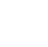Back to Chapter
C++ Setting UpC++ IntroductionNo items found.
C++ BasicsNo items found.
C++ Control StatementsNo items found.
C++ Logical OperatorsNo items found.
C++ Procedural ProgrammingNo items found.
C++ Structural ProgrammingNo items found.
C++ Implementation of OOPSNo items found.
C++ Arrays and VectorsNo items found.
C++ Error HandlingNo items found.
C++ File HandlingNo items found.

# C++ Functions

A Function is a block of code that can be reused many times throughout the program. The idea is to put some commonly or repeatedly done task together and make a function so that instead of writing the same code again and again for different inputs, we can write a single line to call the function which makes our code more compact.

Syntax:

``` return_type function_name([ arg1_type arg1_name, ... ]) { ......... } ```

Functions are further subdivided into user-defined and pre-defined

Let us look into each of them in detail.

### User-defined functions

A user-defined function is a function provided by the user of a program or environment, in a context where the usual assumption is that functions are built into the program or environment. User-defined functions are written by us for our own requirements.

Consider a program to swap two numbers.

``` #include using namespace std; main() { int a, b; cout << "enter a:\n"; cin >> a; cout << "enter b:\n"; cin >> b; int temp = a; //code to swap the numbers a = b; b = temp; cout << "swapped numbers:\na:" << a; cout << "\nb:" << b; } ```
``` Output: enter a: 1 enter b: 5 swapped numbers: a:5 b:1 ```

But what if we had to swap multiple numbers, it would become a tedious job. Instead, we can just put the swapping piece of code in a function, and give it a unique name following the following rules

• Don't use a C++ keyword in your function name.
• They should be lowercase.
• They can have numbers.
• They can be any length (within reason), but keep them short.

And then we pass the numbers to be swapped as actual parameters.

When a function is called, the values (expressions) that are passed in the call are called the arguments or actual parameters (both terms mean the same thing).

``` #include using namespace std; void swap(int & a, int & b) //pass by reference { int temp = a; a = b; b = temp; } main() { int a; int b; cout << "enter a:"; cin >> a; cout << "\nenter b:"; cin >> b; cout << "after swapping:\n"; swap(a, b); cout << "a:" << a << endl; cout << "b:" << b; } ```
``` Output: enter a:1 enter b:4 after swapping: a:4 b:1 ```

### Pass by Value and Pass by Reference

In the above code we are calling the swap function and we are passing the actual arguments by reference.

``` void swap(int &x,int &y) ```

#### call by reference

Recall we learned in C++ data types |&| is used to refer to the address of the variable. To understand what pass by reference is let us execute the same program using pass by value where the actual parameters in the function call are simply copied to the formal parameters.

``` #include using namespace std; void swap(int a, int b)//pass by value { int temp=a; a=b; b=temp; } main() { int a; int b; cout<<"enter a:"; cin>>a; cout<<"\nenter b:"; cin>>b; cout<<"after swapping:\n"; swap(a,b); cout<<"a:"<```
``` Output: enter a: 5 enter b: 1 after swapping: a:5 b:1 ```

`There is no change in the swapped values this is because whatever we performed in the function was applicable only to the formal parameters and not to the actual parameters therefore we need to use the call by reference method when we wish to change the values of the actual parameters.`

`call by value for the swap function`

`The call by reference method of passing parameters to a function copies the reference of an actual parameter into the formal parameter. Inside the function, the reference is used to access the actual parameter used in the call. This means that changes made to the formal parameter affect the passed actual parameter.`

`The call by value method of passing arguments to a function copies the actual value of an argument into the formal parameter of the function`

`‍`

`‍`

`Menu`
`HomeCoursesEventsCampus Ambassador Program`
`About`
`About UsPrivacy PolicyTerms and ConditionsCancellation and Refund PolicyContact UsCareers`
`Courses`
`Full-Stack Web Dev Industrial Training Program`
`Data Scientist Industrial Training Program`
`DevTown Bytes`
`RoadmapsCheatsheetsVideosBlogs`
``` ```
``````
```Ask queriesContact Us on WhatsappHi, How Can We Help You? ```